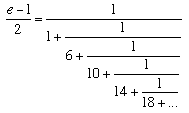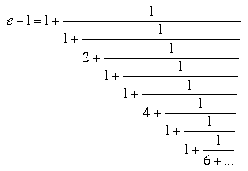# The number e

One of the first articles which we included in the "History Topics" section of our web archive was on the history of π. It is a very popular article and has prompted many to ask for a similar article about the number e. There is a great contrast between the historical developments of these two numbers and in many ways writing a history of e is a much harder task than writing one for π. The number e is, compared to π, a relative newcomer on the mathematics scene.

The number e first comes into mathematics in a very minor way. This was in 1618 when, in an appendix to Napier's work on logarithms, a table appeared giving the natural logarithms of various numbers. However, that these were logarithms to base e was not recognised since the base to which logarithms are computed did not arise in the way that logarithms were thought about at this time. Although we now think of logarithms as the exponents to which one must raise the base to get the required number, this is a modern way of thinking. We will come back to this point later in this essay. This table in the appendix, although carrying no author's name, was almost certainly written by Oughtred. A few years later, in 1624, again e almost made it into the mathematical literature, but not quite. In that year Briggs gave a numerical approximation to the base 10 logarithm of e but did not mention e itself in his work.

The next possible occurrence of e is again dubious. In 1647 Saint-Vincent computed the area under a rectangular hyperbola. Whether he recognised the connection with logarithms is open to debate, and even if he did there was little reason for him to come across the number e explicitly. Certainly by 1661 Huygens understood the relation between the rectangular hyperbola and the logarithm. He examined explicitly the relation between the area under the rectangular hyperbola yx = 1 and the logarithm. Of course, the number e is such that the area under the rectangular hyperbola from 1 to e is equal to 1. This is the property that makes e the base of natural logarithms, but this was not understood by mathematicians at this time, although they were slowly approaching such an understanding.

Huygens made another advance in 1661. He defined a curve which he calls "logarithmic" but in our terminology we would refer to it as an exponential curve, having the form y = kax. Again out of this comes the logarithm to base 10 of e, which Huygens calculated to 17 decimal places. However, it appears as the calculation of a constant in his work and is not recognised as the logarithm of a number (so again it is a close call but e remains unrecognised).

Further work on logarithms followed which still does not see the number e appear as such, but the work does contribute to the development of logarithms. In 1668 Nicolaus Mercator published Logarithmotechnia which contains the series expansion of log(1+x). In this work Mercator uses the term "natural logarithm" for the first time for logarithms to base e. The number e itself again fails to appear as such and again remains elusively just round the corner.

Perhaps surprisingly, since this work on logarithms had come so close to recognising the number e, when e is first "discovered" it is not through the notion of logarithm at all but rather through a study of compound interest. In 1683 Jacob Bernoulli looked at the problem of compound interest and, in examining continuous compound interest, he tried to find the limit of (1 + 1/n)n as n tends to infinity. He used the binomial theorem to show that the limit had to lie between 2 and 3 so we could consider this to be the first approximation found to e. Also if we accept this as a definition of e, it is the first time that a number was defined by a limiting process. He certainly did not recognise any connection between his work and that on logarithms.

We mentioned above that logarithms were not thought of in the early years of their development as having any connection with exponents. Of course from the equation x = at, we deduce that t = log x where the log is to base a, but this involves a much later way of thinking. Here we are really thinking of log as a function, while early workers in logarithms thought purely of the log as a number which aided calculation. It may have been Jacob Bernoulli who first understood the way that the log function is the inverse of the exponential function. On the other hand the first person to make the connection between logarithms and exponents may well have been James Gregory. In 1684 he certainly recognised the connection between logarithms and exponents, but he may not have been the first.

As far as we know the first time the number e appears in its own right is in 1690. In that year Leibniz wrote a letter to Huygens and in this he used the notation b for what we now call e. At last the number e had a name (even if not its present one) and it was recognised. Now the reader might ask, not unreasonably, why we have not started our article on the history of e at the point where it makes its first appearance. The reason is that although the work we have described previously never quite managed to identify e, once the number was identified then it was slowly realised that this earlier work is relevant. Retrospectively, the early developments on the logarithm became part of an understanding of the number e.

We mentioned above the problems arising from the fact that log was not thought of as a function. It would be fair to say that Johann Bernoulli began the study of the calculus of the exponential function in 1697 when he published Principia calculi exponentialium seu percurrentium . The work involves the calculation of various exponential series and many results are achieved with term by term integration.

So much of our mathematical notation is due to Euler that it will come as no surprise to find that the notation e for this number is due to him. The claim which has sometimes been made, however, that Euler used the letter e because it was the first letter of his name is ridiculous. It is probably not even the case that the e comes from "exponential", but it may have just be the next vowel after "a" and Euler was already using the notation "a" in his work. Whatever the reason, the notation e made its first appearance in a letter Euler wrote to Goldbach in 1731. He made various discoveries regarding e in the following years, but it was not until 1748 when Euler published Introductio in Analysin infinitorum that he gave a full treatment of the ideas surrounding e. He showed that
e = 1 + 1/1! + 1/2! + 1/3! + ...
and that e is the limit of (1 + 1/n)n as n tends to infinity. Euler gave an approximation for e to 18 decimal places,
e = 2.718281828459045235
without saying where this came from. It is likely that he calculated the value himself, but if so there is no indication of how this was done. In fact taking about 20 terms of 1 + 1/1! + 1/2! + 1/3! + ... will give the accuracy which Euler gave. Among other interesting results in this work is the connection between the sine and cosine functions and the complex exponential function, which Euler deduced using De Moivre's formula.

Interestingly Euler also gave the continued fraction expansion of e and noted a pattern in the expansion. In particular he gaveusually written 1/1+ 1/6+ 1/10+ 1/14+ 1/18+ ... or [0; 1, 6, 10, 14, ...]
andor [1; 1, 2, 1, 1, 2, 1, 1, 4, 1, 1, 6, ...]
Euler did not give a proof that the patterns he spotted continue (which they do) but he knew that if such a proof were given it would prove that e is irrational. For, if the continued fraction for (e - 1)/2 were to follow the pattern shown in the first few terms, 6, 10, 14, 18, 22, 26, ... (add 4 each time) then it will never terminate so (e - 1)/2 (and so e) cannot be rational. One could certainly see this as the first attempt to prove that e is not rational.

The same passion that drove people to calculate to more and more decimal places of π never seemed to take hold in quite the same way for e. There were those who did calculate its decimal expansion, however, and the first to give e to a large number of decimal places was Shanks in 1854. It is worth noting that Shanks was an even more enthusiastic calculator of the decimal expansion of π. Glaisher showed that the first 137 places of Shanks calculations for e were correct but found an error which, after correction by Shanks, gave e to 205 places. In fact one needs about 120 terms of 1 + 1/1! + 1/2! + 1/3! + ... to obtain e correct to 200 places.

In 1864 Benjamin Peirce had his picture taken standing in front of a blackboard on which he had written the formula i -i = √(eπ). In his lectures he would say to his students:-
Gentlemen, we have not the slightest idea what this equation means, but we may be sure that it means something very important.
Most people accept Euler as the first to prove that e is irrational. Certainly it was Hermite who proved that e is not an algebraic number in 1873. It is still an open question whether ee is algebraic, although of course all that is lacking is a proof - no mathematician would seriously believe that ee is algebraic! As far as we are aware, the closest that mathematicians have come to proving this is a recent result that at least one of ee and e to the power e2 is transcendental.

Further calculations of decimal expansions followed. In 1884 Boorman calculated e to 346 places and found that his calculation agreed with that of Shanks as far as place 187 but then became different. In 1887 Adams calculated the logarithm of e to the base 10 to 272 places.

Anyone wishing to see e to 10,000 places - click THIS LINK.

Article by: J J O'Connor and E F Robertson

List of References (2 books/articles)

 JOC/EFR © September 2001 Copyright information School of Mathematics and Statistics University of St Andrews, Scotland